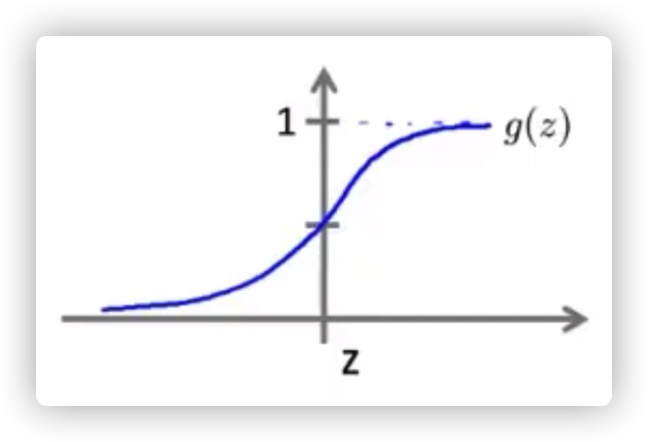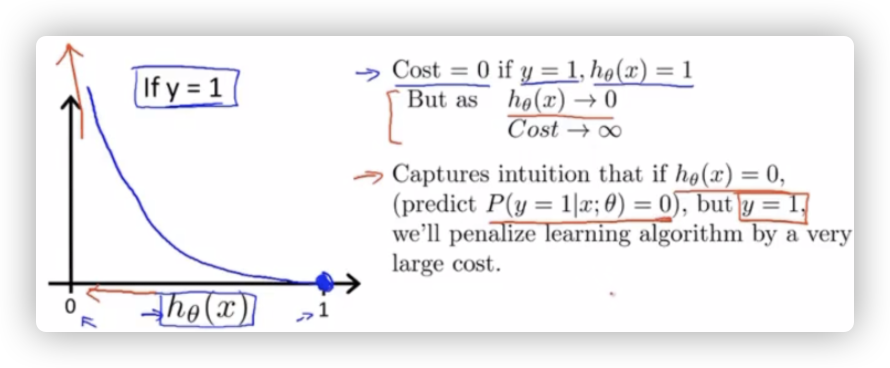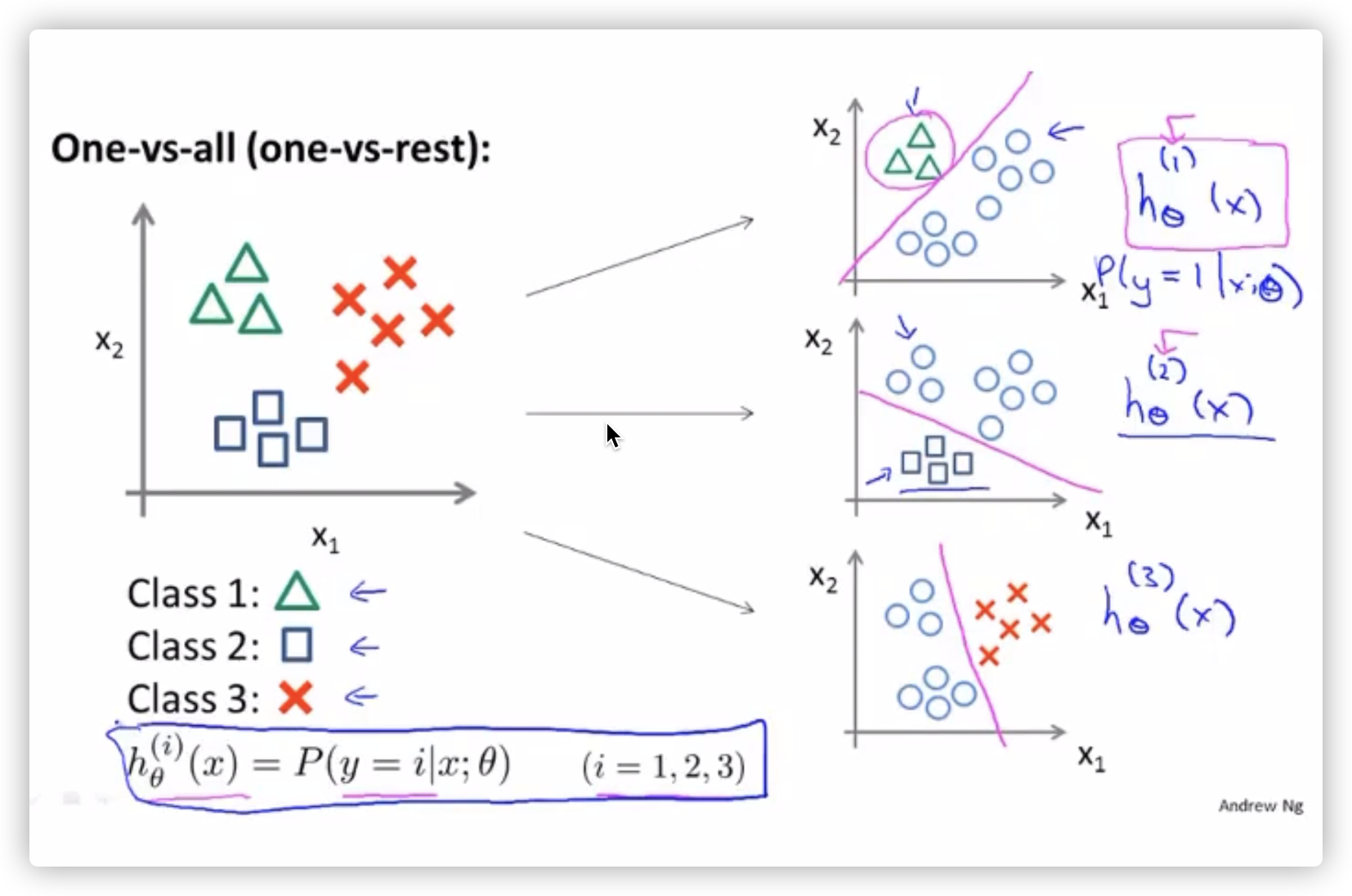# 机器学习整理（逻辑回归）

## 二分类问题

$h(x) = g(\theta^Tx)$

$g(z) = \dfrac{1}{1 +e^{-z}}$$$h(x) >= 0.5$$ 的时候，y 更有可能预测为 1。

$$h(x) < 0.5$$ 的时候，y 更有可能预测为 0。

## 代价函数

$$g(x)$$ 是一个“非凸函数”，如果将点距离公式带入到逻辑回归中，就会存在很多局部最优解$J(\theta) = -\frac{1}{m} [\sum_{i=1}^{m}y_ilog(h(x_i)) + (1-y_i)log(1-h(x_i)) ]$

## 多分类问题